### Triangles - Revision Notes

CBSE Class 09 Mathematics

Revision Notes
CHAPTER – 7
TRIANGLES

1. Congruence of Triangles
2. Criteria for Congruence of Triangles
3. Some Properties of a Triangle
4. Inequalities in a Triangle

• Triangle - A closed figure formed by three intersecting lines is called a triangle. A triangle has three sides, three angles and three vertices.
• Congruent figures - Congruent means equal in all respects or figures whose shapes and sizes both are  same. For example, two circles of the same radii are congruent. Also two squares of the same sides are congruent.
• Congruent Triangles - Two triangles are congruent if and only if one of them can be made to superimpose on the other, so as to cover it completely
• If two triangles ABC and PQR are congruent under the correspondence $A↔P,B↔Q\phantom{\rule{thickmathspace}{0ex}}and\phantom{\rule{thinmathspace}{0ex}}C↔R$ then symbolically, it is expressed as $\mathrm{\Delta }ABC\cong \phantom{\rule{thickmathspace}{0ex}}\mathrm{\Delta }PQR$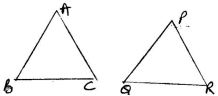• In congruent triangles, corresponding parts are equal and we write 'CPCT' for corresponding parts of congruent triangles.
• SAS congruency rule - Two triangles are congruent if two sides and the included angle  between two sides of one triangle are equal to the two sides and the included angle between two sides  of the other triangle. For example $\mathrm{\Delta }ABC$ and $\phantom{\rule{thickmathspace}{0ex}}\mathrm{\Delta }PQR$ as shown in the figure satisfy SAS congruence criterion.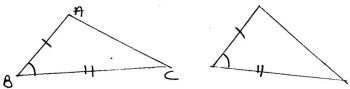• ASA Congruence Rule - Two triangles are congruent if two angles and the included side of one triangle are equal to two angles and the included side of other triangle. For examples $\mathrm{\Delta }ABC\phantom{\rule{thickmathspace}{0ex}}and\phantom{\rule{thickmathspace}{0ex}}\mathrm{\Delta }DEF$ shown below satisfy ASA congruence criterion.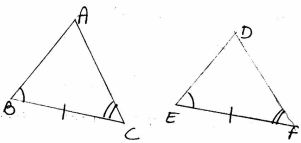• AAS Congruence Rule - Two triangle are congruent if any two pairs of angles and one pair of corresponding sides are equal.For example $\mathrm{\Delta }ABC\phantom{\rule{thickmathspace}{0ex}}and\phantom{\rule{thickmathspace}{0ex}}\mathrm{\Delta }DEF$ shown below satisfy AAS congruence criterion.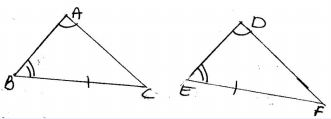• AAS criterion for congruence of triangles is a particular case of ASA criterion.
• Isosceles Triangle - A triangle in which two sides are equal is called an isosceles triangle. For example $\mathrm{\Delta }ABC$ shown below is an isosceles triangle with AB=AC.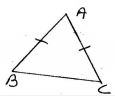• Scalene Triangle - A triangle, no two of whose sides are equal, is called scalene triangle.
• Equilateral Triangle - A triangle whose all sides are equal, is calle an equilateral triangle.
• Right angled triangle - A triangle with one right angle is called a right angled triangle.
• The sum of all the angles of a triangle is 180o.
• If a side of a triangle is produced, the exterior angle so formed is equal to the sum of two interior opposite angles.
• Angle opposite to equal sides of a triangle are equal.
• Sides opposite to equal angles of a triangle are equal.
• Each angle of an equilateral triangle is ${60}^{o}$.
• If the altitude from one vertex of a triangle bisects the base, then the triangle is isosceles triangle.
• SSS congruence Rule - If three sides of one triangle are equal to the three sides of another triangle then the two triangles are congruent for example $\mathrm{\Delta }ABC\phantom{\rule{thickmathspace}{0ex}}and\phantom{\rule{thickmathspace}{0ex}}\mathrm{\Delta }DEF$ as shown in the figure satisfy SSS congruence criterion.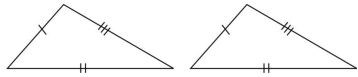• RHS Congruence Rule - If in two right triangles the hypotenuse and one side of one triangle are equal to the hypotenuse and one side of the other triangle then the two triangle are congruent. For example $\mathrm{\Delta }ABC\phantom{\rule{thickmathspace}{0ex}}and\phantom{\rule{thickmathspace}{0ex}}\mathrm{\Delta }PQR$ shown below satisfy RHS congruence criterion.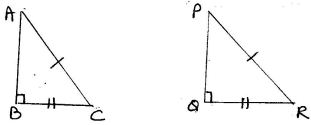RHS stands for Right angle - Hypotenuse side.
• A point equidistant from two given points lies on the perpendicular bisector of the line segment joining the two points and vice-versa.
• A point equidistant from two intersecting lines lies on the bisectors of the angles formed by the two lines.
• In a triangle, angle opposite to the longer side is larger (greater)
• In a triangle, side opposite to the larger (greater) angle is longer.
• Sum of any two sides of a triangle is greater than the third side.